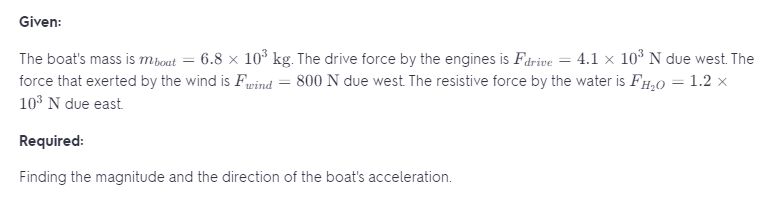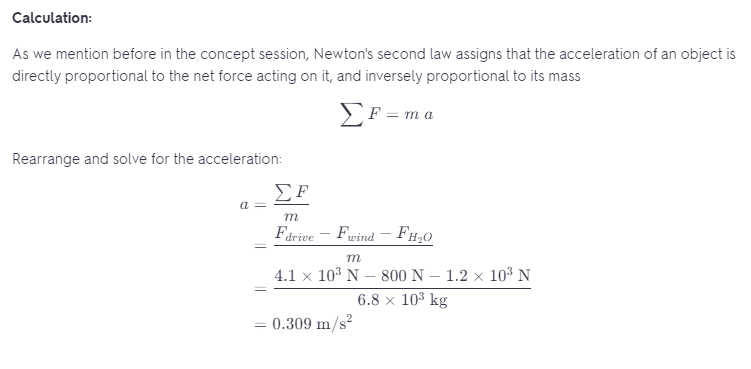# A Boat Has A Mass Of 6800 Kg

We thoroughly check each answer to a question to provide you with the most correct answers. Found a mistake? Let us know about it through the REPORT button at the bottom of the page.

A boat has a mass of 6800 kg. Its engines generate a drive force of 4100 N, due west, while the wind exerts a force of 800 N, due east, and the water exerts a resistive force of 1200 N due east. What is the magnitude and direction of the boat’s acceleration?

a = 0.309 m/s^2

As we know the core of classical mechanics is Newton’s three laws of motion which are the basic classical laws describing motion. As we know Newton’s first law assigns that if the net force on an object s zero, an object originally at rest remains at rest, and an object in motion remains in motion in a straight line with constant velocity.

Also, we know that Newton’s second law assigns that the acceleration of an object is directly proportional to the net force acting on it, and inversely proportional to its mass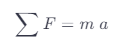As we know Newton’s third law assigns that whenever one object exerts a force on a second object, the second object always exerts a force on the first object which is equal in magnitude but opposite in direction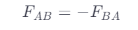As we know the weight refers to the gravitational force on an object, and is equal to the product of the object’s mass \$m\$ and the acceleration of gravity g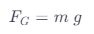The force which is a vector, can be considered as a push or pull. As we know the friction that object exerts on the other can be written as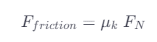Where Fn is the normal force (the force each object exerts on the perpendicular to their constant surfaces).